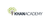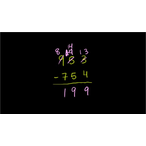# Class 11 Physics (India): Bernoulli's equation (part 2)

Type product
Tijdsduur

## Class 11 Physics (India): Bernoulli's equation (part 2)Khan Academy

Tip: laat jezelf inspireren, bekijk hier de video!### Beschrijving

This is the second of two videos where Sal derives Bernoulli's equation. In the second half of the video Sal also begins an example problem where liquid exits a hole in a container.

## Class 11 Physics (India)

Let's learn, practice, and master topics of class 11 physics (NCERT) starting with kinematics and then moving to dynamics with Newton's laws of motion, work, energy, and power. Let's then use these as the foundation to learn about centre of mass, rotational motion, gravitation, solids, fluids, thermodynamics, and oscillations and waves.

## Fluid dynamics

Flowing fluids behave different from static fluids. In these videos and articles you'll learn about volume flow rate and the equation of co…

Lees de volledige beschrijving

### Veelgestelde vragen

Er zijn nog geen veelgestelde vragen over dit product. Als je een vraag hebt, neem dan contact op met onze klantenservice.

Nog niet gevonden wat je zocht? Bekijk deze onderwerpen: Natuurkunde, Biologie, Chemie, Wildbeheer en Assessment.

This is the second of two videos where Sal derives Bernoulli's equation. In the second half of the video Sal also begins an example problem where liquid exits a hole in a container.

## Class 11 Physics (India)

Let's learn, practice, and master topics of class 11 physics (NCERT) starting with kinematics and then moving to dynamics with Newton's laws of motion, work, energy, and power. Let's then use these as the foundation to learn about centre of mass, rotational motion, gravitation, solids, fluids, thermodynamics, and oscillations and waves.

## Fluid dynamics

Flowing fluids behave different from static fluids. In these videos and articles you'll learn about volume flow rate and the equation of continuity. You'll also learn how Bernoulli's principle and Bernoulli's equation relate the pressure and speed of a flowing fluid. The effects of turbulence and viscosity will also be discussed.

## Topic: Science

Learn about all the sciences, from physics, chemistry and biology, to cosmology and astronomy, across hundreds of videos, articles and practice questions.

Er zijn nog geen ervaringen.
• Bekijk gerelateerde producten mét ervaringen: Natuurkunde.

### Deel je ervaring

Heb je ervaring met deze cursus? Deel je ervaring en help anderen kiezen. Als dank voor de moeite doneert Springest € 1,- aan Stichting Edukans.

Er zijn nog geen veelgestelde vragen over dit product. Als je een vraag hebt, neem dan contact op met onze klantenservice.